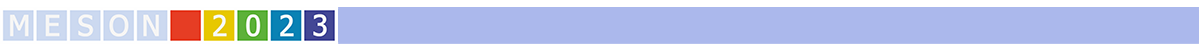#MESON2023

Jun 22 – 27, 2023
Auditorium Maximum
Europe/Warsaw timezone

## Xi nuclear constraints from recent Xi^- capture events in emulsion

Jun 26, 2023, 3:00 PM
25m
Medium lecture hall (B) (Auditorium Maximum)

Invited parallel

### Speaker

Avraham Gal (Hebrew University)

### Description

All five KEK and J-PARC two-body $\Xi^-$+$^A$Z $\to$ twin-$\Lambda$ capture events in light emulsion nuclei are consistent with Coulomb-assisted $1p_{\Xi^-}$ nuclear states. The underlying $\Xi$-nuclear potential is strongly attractive with nuclear-matter depth $V_{\Xi}\geq$ 20 MeV , considerably larger than suggested by recent LQCD, femtoscopy and EFT theoretical studies.
We argue that the J-PARC E07 new $^{14}$N capture events  assigned to $1s_{\Xi^-}$ nuclear states, thereby implying considerably shallower $V_{\Xi}$, have also another interpretation as $1p_{\Xi^0}$ nuclear states .

 E. Friedman, A. Gal, Constraints on $\Xi^-$ nuclear interactions from capture events in emulsion, Phys. Lett. B 820,136555 (2021).

 M. Yoshimoto et al. (J-PARC E07 Collab.), First observation of a nuclear $s$-state of $\Xi$ hypernucleus, ${_{\Xi}^{15}}$C, Prog. Theor. Exp. Phys. 2021, 073D02 (2021).

 E. Friedman, A. Gal, Has J-PARC E07 observed a $\Xi^-_{1s}$ nuclear state?, Phys. Lett. B 837, 137640 (2022).

### Primary author

Avraham Gal (Hebrew University)

### Co-author

Eliahu Friedman (Hebrew University)